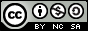LeetCode 392 判断子序列(dp)

**示例 1:**s = "abc", t = "ahbgdc"

**示例 2:**s = "axc", t = "ahbgdc"

## 代码

  1 2 3 4 5 6 7 8 9 10 11 12 13 14 15 16  class Solution { public: bool isSubsequence(string s, string t) { int i = 0, j = 0; while (i < s.size() && j < t.size()) { if (s[i] == t[j]) i++, j++; else j++; } return i == s.size(); } }; 

dp代码：

  1 2 3 4 5 6 7 8 9 10 11 12 13 14 15 16 17 18 19 20 21 22 23 24 25 26 27  class Solution { public: bool isSubsequence(string s, string t) { int n = s.size(), m = t.size(); vector> dp(m + 1, vector(26, 0)); for (int i = 0; i < 26; i++) dp[m][i] = m; for (int i = m - 1; i >= 0; i--) for (int j = 0; j < 26; j++) if (t[i] == j + 'a') dp[i][j] = i; else dp[i][j] = dp[i + 1][j]; int i = 0; for (int j = 0; j < n; j++) { if (dp[i][s[j] - 'a'] == m) return false; i = dp[i][s[j] - 'a'] + 1; } return true; } };